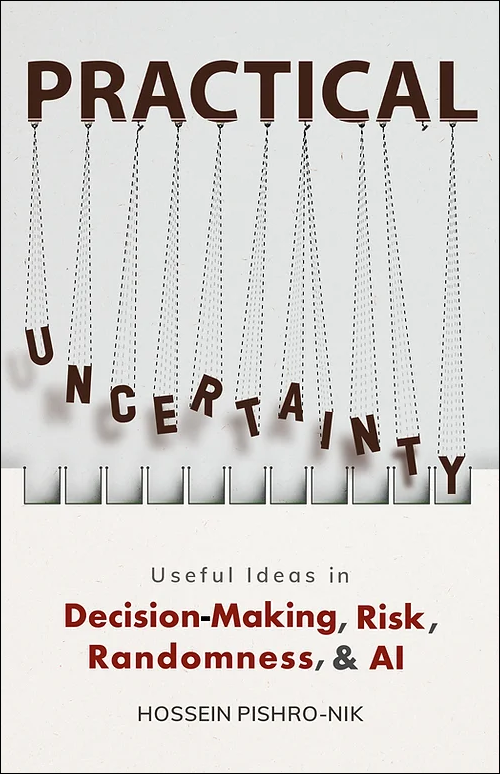## 1.2 Review of Set Theory

Probability theory uses the language of sets. As we will see later, probability is defined and calculated for sets. Thus, here we briefly review some basic concepts from set theory that are used in this book. We discuss set notations, definitions, and operations (such as intersections and unions). We then introduce countable and uncountable sets. Finally, we briefly discuss functions. This section may seem somewhat theoretical and thus less interesting than the rest of the book, but it lays the foundation for what is to come.

A set is a collection of some items (elements). We often use capital letters to denote a set. To define a set we can simply list all the elements in curly brackets, for example to define a set $A$ that consists of the two elements $\clubsuit$ and $\diamondsuit$, we write $A=\{\clubsuit,\diamondsuit\}$. To say that $\diamondsuit$ belongs to $A$, we write $\diamondsuit \in A$, where "$\in$" is pronounced "belongs to." To say that an element does not belong to a set, we use $\notin$. For example, we may write $\heartsuit \notin A$.

A set is a collection of things (elements).

Note that ordering does not matter, so the two sets $\{\clubsuit,\diamondsuit\}$ and $\{\diamondsuit,\clubsuit\}$ are equal. We often work with sets of numbers. Some important sets are given the following example.

Example
The following sets are used in this book:

• The set of natural numbers, $\mathbb{N}=\{1,2,3,\cdots\}$.
• The set of integers, $\mathbb{Z}=\{\cdots,-3,-2,-1,0,1,2,3,\cdots\}$.
• The set of rational numbers $\mathbb{Q}$.
• The set of real numbers $\mathbb{R}$.
• Closed intervals on the real line. For example, $[2,3]$ is the set of all real numbers $x$ such that $2\leq x\leq3$.
• Open intervals on the real line. For example $(-1,3)$ is the set of all real numbers $x$ such that $-1< x <3$.
• Similarly, $[1,2)$ is the set of all real numbers $x$ such that $1\leq x <2$.
• The set of complex numbers $\mathbb{C}$ is the set of numbers in the form of $a+bi$, where $a,b \in \mathbb{R}$, and $i=\sqrt{-1}$.

We can also define a set by mathematically stating the properties satisfied by the elements in the set. In particular, we may write

$A=\{x | x \textrm{ satisfies some property} \}$
or
$A=\{x : x \textrm{ satisfies some property} \}$

The symbols $"|"$ and $":"$ are pronounced "such that."

Example
Here are some examples of sets defined by stating the properties satisfied by the elements:

• If the set $C$ is defined as $C=\{x | x \in \mathbb{Z}, -2 \leq x < 10\}$, then $C=\{-2,-1,0,\cdots,9\}$.
• If the set $D$ is defined as $D=\{x^2 |x\in \mathbb{N}\}$, then $D=\{1,4,9,16,\cdots\}$.
• The set of rational numbers can be defined as $\mathbb{Q}=\{\frac{a}{b}|a,b \in \mathbb{Z}, b \neq 0\}$.
• For real numbers $a$ and $b$, where $a < b$, we can write $(a,b]=\{x \in \mathbb{R} \mid a< x \leq b \}$.
• $\mathbb{C}=\{a+bi \mid a,b \in \mathbb{R}, i=\sqrt{-1}\}$.

Set $A$ is a subset of set $B$ if every element of $A$ is also an element of $B$. We write $A \subset B$, where "$\subset$" indicates "subset." Equivalently, we say $B$ is a superset of $A$, or $B \supset A$.

Example
Here are some examples of sets and their subsets:

• If $E=\{1,4\}$ and $C=\{1,4,9\}$, then $E\subset C$.
• $\mathbb{N}\subset \mathbb{Z}$.
• $\mathbb{Q}\subset \mathbb{R}$.

Two sets are equal if they have the exact same elements. Thus, $A=B$ if and only if $A\subset B$ and $B\subset A$. For example, $\{1,2,3\}=\{3,2,1\}$, and $\{a,a,b\}=\{a,b\}$. The set with no elements, i.e., $\emptyset=\{ \}$ is the null set or the empty set. For any set $A$, $\emptyset \subset A$.

The universal set is the set of all things that we could possibly consider in the context we are studying. Thus every set $A$ is a subset of the universal set. In this book, we often denote the universal set by $S$ (As we will see, in the language of probability theory, the universal set is called the sample space.) For example, if we are discussing rolling of a die, our universal set may be defined as $S=\{1,2,3,4,5,6\}$, or if we are discussing tossing of a coin once, our universal set might be $S=\{H,T\}$ ($H$ for heads and $T$ for tails).

 The print version of the book is available on Amazon.Practical uncertainty: Userful Ideas in Decision-Making, Risk, Randomness, & AI